Next: 4.4.1 Vortex Lattice in Up: 4 Modelling the Internal Previous: 4.3 Gaussian Field Distribution

# 4.4 Field Distribution of the Vortex Lattice

A more appropriate way of analyzing the measured field distribution is to model the contributions to the line shape separately. The London theory provides the simplest approach to modelling the internal field distribution n(B) corresponding to the vortex lattice. The London model applies exclusively to extreme type II superconductors in which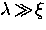, and is independent of the detailed mechanism responsible for superconductivity. Furthermore, the London picture is valid at all temperatures below Tc and for applied magnetic fields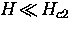. Both of these conditions are usually satisfied in a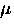SR experiment.

For a magnetic field applied in the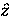-direction parallel to the crystallographic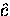-axis, the London equation [i.e. Eq. (2.13)] for the field profile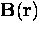resulting from vortices positioned at the sites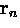is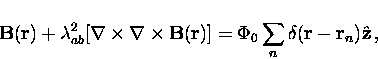(6)
where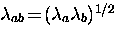,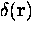is a two-dimensional delta function and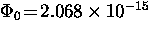T-m2 is the flux associated with each vortex. We restrict the discussion throughout this report to the above mentioned orientation between the applied magnetic field and the crystal lattice--hence avoiding generalizing equations to include the-axis penetration depth. The pointsform a two-dimensional periodic lattice in the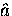-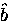plane, so thatmay be expanded in a Fourier series. The Fourier transform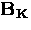is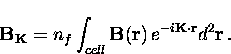(7)
Here nf is the number of vortices per unit area and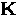are the reciprocal lattice vectors. From Eq. (4.6) the Fourier components are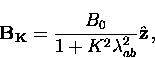(8)
where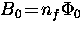is the average internal field. Thus the total field at the point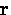is given by

Eq. (4.9) does not account for the finite size of the vortex cores, which are assumed to be infinitely small--i.e. a delta function is used as the source term in Eq. (4.6). The spatial dependence of the order parameter which goes to zero at the center of the vortex cores is not built into the London theory. As a result Eq. (4.9) has the unphysical property thatdiverges on the axis of the vortex line at. To correct for the finite size of the vortex cores, one can modify Eq. (4.9) by multiplying each term by a cutoff factor which suppresses the higher Fourier components and produces a smooth variation of field to a finite maximum value at the center of the vortex core. A sharp cutoff such as at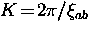is generally inappropriate because it introduces an oscillatory cutoff in real space . A smooth cutoff may be obtained by solving the GL equations. At reduced fields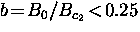,Brandt  derived the cutoff factor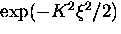from the isotropic GL theory for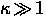. At low fields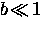, the cutoff factor is better approximated by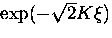. To account for the field dependence of the order parameter in GL theory, Brandt also replaces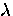and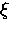with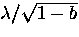and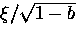, respectively. With the external magnetic field applied parallel to the crystallographic-axis, the local field at any point in the-plane is then given by Brandt's modified London model [54,122,123]

where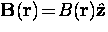and the cutoff factor should be replaced with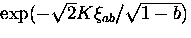when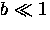.In Chapter 6, we shall show that Eq. (4.10) is a reasonable model for the internal field distribution due to the vortex lattice in a real superconductor.Next: 4.4.1 Vortex Lattice in Up: 4 Modelling the Internal Previous: 4.3 Gaussian Field Distribution